# RD Sharma Solutions for Class 8 Math Chapter 6 - Algebraic Expressions and Identities (Part-1) Notes | Study RD Sharma Solutions for Class 8 Mathematics - Class 8

## Class 8: RD Sharma Solutions for Class 8 Math Chapter 6 - Algebraic Expressions and Identities (Part-1) Notes | Study RD Sharma Solutions for Class 8 Mathematics - Class 8

The document RD Sharma Solutions for Class 8 Math Chapter 6 - Algebraic Expressions and Identities (Part-1) Notes | Study RD Sharma Solutions for Class 8 Mathematics - Class 8 is a part of the Class 8 Course RD Sharma Solutions for Class 8 Mathematics.
All you need of Class 8 at this link: Class 8

#### Question 1: Identify the terms, their coefficients for each of the following expressions:

(i) 7x2yz − 5xy
(ii) x2 + x + 1
(iii) 3x2y2 − 5x2y2z2 + z2
(iv) 9 − ab + bc − ca

(v) a/2 + b/2 -ab

(vi) 0.2 0.3xy + 0.5y

term in an algebraic expression can be a constant, a variable or a product of constants and variables separated by the signs of addition (+) or subtraction (-) . Examples: 27, xxyz,1/2x2y12x2yzetc.

The number factor of the term is called its coefficient.

(i) The expression 7x2yz5xy7x2yz-5xy consists of two terms, i.e.,  7x2yz7x2yz and 5xy-5xy.
The coefficient of 7x2yz7x2yz is 7 and the coefficient of 5xy-5xy is 5-5.
(ii) The expression x2+x+1x2+x+1 consists of three terms , i.e., x2x2x and 1.
The coefficient of each term is 1.
(iii) The expression 3x2y25x2y2z2+z23x2y2-5x2y2z2+z2 consists of three terms , i.e., 3x2y23x2y25x2y2z2-5x2y2z2 and  z2z2. The coefficient of 3x2y23x2y2 is 3. The coefficient of 5x2y2z2-5x2y2z2 is 5-5 and the coefficient of z2z2 is 1.
(iv) The expression 9ab+bcca9-ab+bc-ca consists of four terms — 9,ab, bc and ca9,-ab, bc and -ca. The coefficient of the term 9 is 9. The coefficient of ab-ab is -1. The coefficient of bcbc is 1, and the coefficient of ca-ca is -1.

(v) The expression a/2 + b/2 -abconsists of three terms  , i.e.,a/2, b/2 and aba2, b2 and -ab. The coefficient of a/2a2 is 1/2. The coefficient of b/2 is (vi) The expression 0.2x0.3xy+0.5y 0.2x-0.3xy+0.5y consists of three terms , i.e., 0.2x, 0.3xy and 0.5y.0.2x, -0.3xy and 0.5y. The coefficient of 0.2x0.2x is 0.20.2. The coefficient of 0.3xy-0.3xy is 0.3-0.3, and the coefficient of 0.5y0.5y is 0.50.5. 1/212, and the coefficient of ab-ab is -1.

#### Question 2: Classify the following polynomials as monomials, binomials, trinomials. Which polynomials do not fit in any category?(i) x + y(ii) 1000(iii) x + x2 + x3 + 4y4(iv) 7 + a + 5b(v) 2b − 3b2(vi) 2y − 3y2 + 4y3(vii) 5x − 4y + 3x(viii) 4a − 15a2(ix) xy + yz + zt + tx(x) pqr(xi) p2q + pq2(xii) 2p + 2q

A polynomial is monomial if it has exactly one term. It is called binomial if it has exactly two non-zero terms. A polynomial is a trinomial if it has exactly three non-zero terms.
(i)   The polynomial x+yx+y has exactly two non zero terms , i.e., x and y. Therefore, it is a binomial.
(ii)  The polynomial 1000 has exactly one term, i.e., 1000. Therefore, it is a monomial.
(iii) The polynomial x+x2+x3+x4x+x2+x3+x4 has exactly four terms, i.e., x, x2, x3 and x4x, x2, x3 and x4. Therefore, it doesn't belong to any of the categories.
(iv) The polynomial 7+a+5b7+a+5b has exactly three terms, i.e., 7, and 5b. Therefore, it is a trinomial.
(v)  The polynomial 2b3b22b-3b2 has exactly two terms, i.e., 2b and 3b2-3b2. Therefore, it is a binomial.
(vi) The polynomial 2y3y2+4y32y-3y2+4y3 has exactly three terms, i.e., 2y, 3y2-3y2 and 4y34y3. Therefore, it is a trinomial.
(vii) The polynomial 5x4y+3x5x-4y+3x has exactly three terms, i.e., 5x-4y and 3x. Therefore, it is a trinomial.
(viii) The polynomial 4a15a24a-15a2 has exactly two terms, i.e., 4a and 15a2-15a2. Therefore, it is a binomial.
(ix) The polynomial xy+yz+zt+txxy+yz+zt+tx has exactly four terms xyyzzt and tx. Therefore, it doesn't belong to any of the categories.
(x) The polynomial pqr has exactly one term, i.e., pqr. Therefore, it is a monomial.
(xi) The polynomial p2q+pq2p2q+pq2 has exactly two terms, i.e., p2qp2q and pq2pq2. Therefore, it is a binomial.
(xi) The polynomial 2p+2q2p+2q has two terms, i.e., 2p and 2q. Therefore, it is a binomial.

#### Question 3: Add the following algebraic expressions:

(i) 3a2b, − 4a2b, 9a2b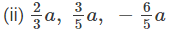(iii) 4xy2 − 7x2y, 12x2y − 6xy2, − 3x2y +5xy2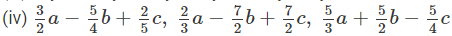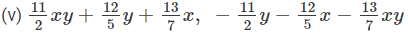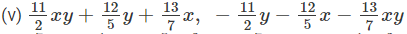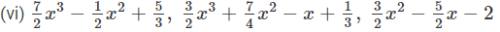#### (v) To add, we proceed as follows: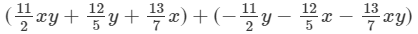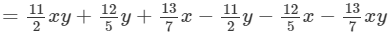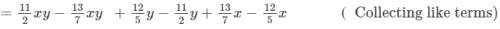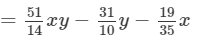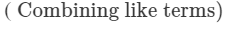(vi) To add, we proceed as follows: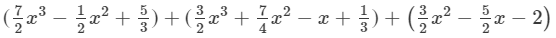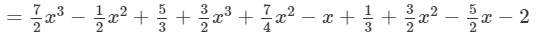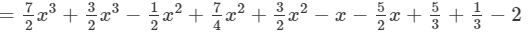( Combining like terms)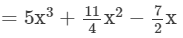( Combining like